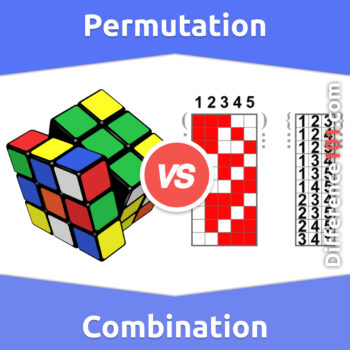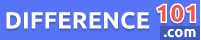# Permutation vs. Combination: What’s The Difference Between Permutation And Combination?

What is the difference between a permutation and a combination? We will explain these two terms and give examples of each. Learn more about their differences and how they are used in mathematics.Permutation vs. Combination: Almost all of us have heard these two terms, permutation, and combination, in Mathematics. These terms may be used together but are different from each other. The main difference between permutation and combination is that permutation is all about the order of objects, while the combination is about selecting the right elements to form the collection.

Let’s take a closer look at Combination vs. Permutation

## What Is Permutation?If we look at the permutation meaning, it is a process of selection in which all cares about the order. In other words, a permutation is a number of ways in which a few or all members are arranged in a particular order. For example, arrangements of digits, alphabets, and numbers properly. A permutation is divided into two different types. The first one is the “Permutation with Repetition”, which means if there is no possibility for the first selection process, then there will be no possibility for the second selection and so on. The second type is “Permutation without repetition”; in this type of permutation, no repetition and order matters.

👉  Cladogram vs. Phylogenetic Tree: What’s The Difference Between Cladogram and Phylogenetic Tree?

## What is Combination?If we look at combination meaning: it is a way of selecting specific items from a given collection. In combination, the order of the selection does not matter. The combination can also refer to a combination of specific items from a group at a time without repetition. Here is a simple example to explain the combination: we have three digits (4,5,6), and we want to make a three-digit number from the given numbers; there are various possibilities of the combination, which are 456, 564, 654, 546, 645, and so on. Combination basically provides information about an easy way to work out how many ways 4, 5, and 6 can be placed in an order.

👉  Semrush vs. SE Ranking: Everything You Need To Know About The Difference Between Semrush And Seranking

## Permutation vs. Combination Similarities

• Whether it is permutation or combination, both are related to each other. As permutation is counting, the number of arrangements and combinations is counting the selection.
• Both of these concepts are used in Mathematics, statistics, research and our daily life as well.
👉  Wii vs. Wii U: What Is The Difference Between Wii And Wii U?

## Permutation vs. Combination Examples

### Permutation Examples

• Picking the winner, first, second and third
• Arranging the digits, alphabets, numbers or letters.
• Picking two favorite colors in order from a coloring book.

### Combination Examples

• Selecting team members from a particular group
• Picking three winners only.
• Selecting the menu, food, or clothes.

## Permutation Vs. Combination Pros and Cons

### Permutation Pros and Cons#### Pros of Permutation

• A permutation is very important in many different ways when it comes to counting problems and other areas of mathematics.
• A permutation is also used in real life, like arranging people, letters, colors, numbers and alphabets.

#### Cons of Permutation

• A permutation is often confused with a combination, as these terms refer to arranging.
• Permutation can be difficult for new students, as it has further types which can be mixed with one another.

### Combination Pros and Cons#### Pros of Combination

• The combination is a mathematical technique which is important to determine the number of possible arrangements in the given items.
• The combination is also used in daily life; for example, in an exam paper, there are options for solving a few questions or a large set of questions.

#### Cons of Combination

• The combination is often confused with permutation, as both terms refer to arranging.
• Permutation can be difficult for new students, as it has further types which can be mixed with one another.

## Comparison Chart## Conclusion

Permutation and combination are the two concepts which we often hear of in mathematics and statistics. These concepts are also used in our day-to-day life as well. But if we talk about permutation vs. Combination differences, both concepts are different from each other. A permutation is basically about the arrangement of the objects, while a combination is all about the selection of a particular object from the group. A permutation is basically a count of different arrangements made from a given set. For example, we have three characters F, 5, \$, and different passwords can be formed by using these numbers, like F5\$, \$5F, 5\$F, and \$F5. While the combination is all about arrangement without concern about an order, for example, the number of different groups can be created from the combination of the available things. If we compare permutation versus combination importance, both are important in mathematics as well as daily life.Alex Stantor

Alex Stantor is a Sorbonne University (Paris, France) graduate in Philosophy and Data Analysis. Currently, he is an Author and Researcher at Difference 101, he writes articles/blog posts on topics such as "thinking differently" and "the importance of difference". Alex is a passionate advocate of diversity in the workplace and in companies, and diversity and inclusion in corporate communications. He currently lives in Brooklyn.

We will be happy to hear your thoughts